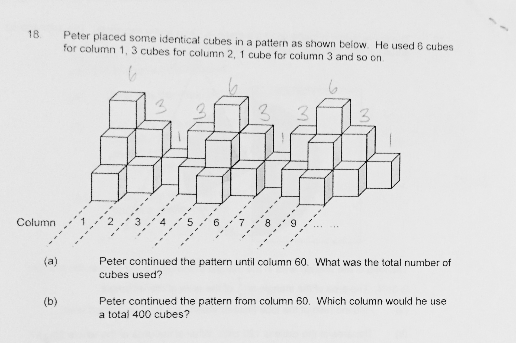# QuestionAnyone help me with attached question

Thanks

The pattern repeats after every 4 columns.

He used a total of 6 + 3 + 1 + 3 = 13 cubes every 4 columns.

(a) 60 ÷ 4 = 15 repetitions

15 × 13 = 195 cubes

(b) 400 ÷ 13 = 30 repetitions + 10 cubes

30 × 4 = 120 columns (390 cubes)

Column 121 : 6 cubes (391 – 396)

Column 122: 3 cubes (397 – 399)

Column 123: 1 cube (400)

— thank you SAHMom!

Per set of patterns consists of 4 columns of 13 cubes.

60/4=15 sets (of 4 columns)

15×13=195 cubes#

400/13 = 30 sets (of 4 columns) + remainder 10 cubes (of 6+3+1 cubes)

30×4 + 3 = 123 columns#

0 Replies 1 Like

(a)
column (1 + 2 + 3) ——- 6 + 3 + 1 = 10
60/3 = 20
20 x 10 = 200

(b)
400/10 = 40
40 x 3 = 120

Ans : (a) 200 cubes; (b) 120 columns.

0 Replies 1 Like